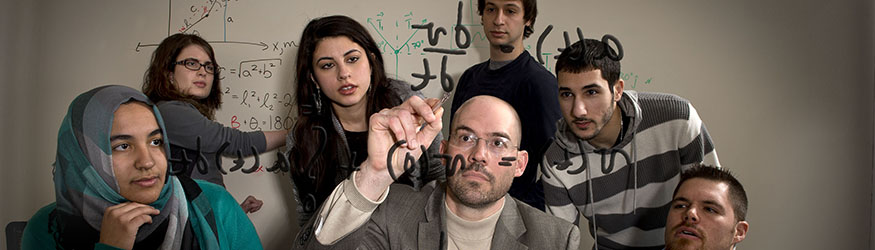# Engineering Mathematics (EGR 1010) Topics and MaterialsEGR 1010 is a mathematics course taught by the College of Engineering and Computer Science faculty, consisting of lecture, lab, and recitation. All topics are driven by engineering applications taken directly from core engineering courses. The lectures are motivated by hands-on laboratory exercises including a thorough integration with Matlab.

## Topics

• Basic Algebraic Manipulations (1.0 weeks)
• Trigonometry (1.0 weeks)
• 2-D Vectors (1.0 weeks)
• Complex Numbers (1.0 weeks)
• Sinusoids & Harmonic Signals (1.0 weeks)
• Matrices & Systems of Equations (1.0 weeks)
• Basics of Differentiation (3.0 weeks)
• Basics of Integration (3.0 weeks)
• Differential Equations (3.0 weeks)

## Sample Materials

(Updated 05/28/2020)

### Homework

Homework is assigned on a weekly basis from the course textbook (5-6 problems) and the course programming book (3-4 problems).

The following topics are covered in this class:

### Laboratory Assignments

There are 8 hands-on lab assignments that supplement the course material. Additionally, there are 4 Matlab assignments that illustrate a more application-based approach to coding.

#### Lab #1

Application of Algebra in Engineering - The One Loop Circuit

#### Lab #2

Trigonometry Relationships - One and Two Link Planar Robots

#### Lab #3

Sinusoids in Engineering - Measurement and Analysis of Harmonic Signals

#### Lab #4

Systems of Engineering - The Two Loop Circuit

#### Lab #5

Derivatives in Engineering - Velocity and Acceleration in Free Fall

#### Lab #6

Integrals in Engineering - Work and Stored Energy in a Spring

#### Lab #7

Differential Equations in Engineering - The Leaking Bucket

#### Lab #8

Differential Equations in Engineering - Spring Mass Vibration